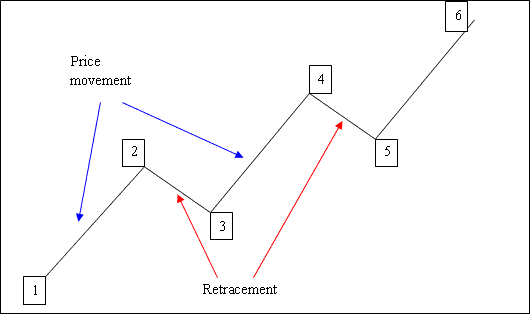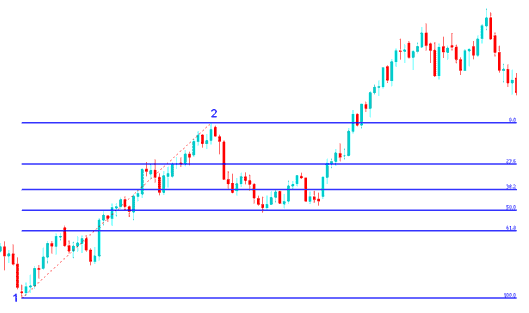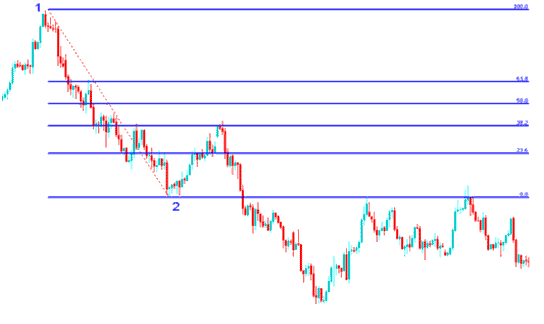# Drawing Fibonacci Retracement Levels on Upward and Downward Gold Trend

The price of a Gold metal does not move up or down in a straight line. Instead price of Gold moves up or down in a zigzag pattern. Fibonacci Retracement is the tool used to calculate where the zigzag will stop. The pullback levels are 38.2%, 50% and 61.8%. These form the points at which the market is likely to make a retracement.

What is a retracement? A retracement is a pullback of the price before the market resumes the original trend/original direction of movement.
Example of Zigzag Movement: The Example below shows price moving up in a zigzag pattern.

The diagram below shows this movement in an upward trending market.1-2: Price moves up
2-3: Pullback
3-4: Moves up
4-5: Pullback
5-6: Moves up

Since we can spot where a pullback starts on a Gold price chart, how do we know where these pullbacks/retracements will reach?
The answer is we use Fibonacci retracement levels

This is a type of line study used in Gold trading to predict and calculate the price retracement levels. This indicator is placed directly on the price chart within the trading platform provided by your online broker, This Fibonacci retracement levels indicator will then automatically calculate these retracement levels on the chart.

What are the Fibonacci Retracement Levels?

23.6 % Fibonacci retracement level
38.2 % Fibonacci retracement level
50.0 % Fibonacci retracement level
61.8 % Fibonacci retracement level

38.2 % and 50.0 % Retracement Levels are the most commonly used and most of the time this is where the pullback will reach - with 38.2 % Fibonacci retracement level being the most popular and the most widely used.

61.8% Fibonacci retracement level is also commonly used to set stops for trades opened using this strategy - because price retracement will not get to this retracement level most of the time.

This Fibonacci retracement levels tool will be drawn in the direction of the Gold price trend as explained in the examples below.

## How to Draw Fibonacci Retracement Levels on an Upward Market Trend

In the diagram below the Gold price is moving up between 1 and 2 then after 2 it retraces down to 50.0% Fibonacci retracement level pull back area then it continues moving up in the original upward trend. Notice that this indicator is drawn from point 1 to point 2 in the direction of the Gold price trend (Upward trend).

Because we know this is just a retracement/pullback based on our using this indicator - Fibonacci retracement level, we put a buy order just between the levels 38.2% and 50.0% retracement levels and our stop loss just below 61.8% pull back level. If you had put a buy at this point in the trade example below you would have made a lot of pips as shown below.Fibonacci Retracement Levels Drawn on an Upward Gold Price Trend## Explanation for the Above Fibonacci Retracement Setup Trade Example

Once the Gold price hit the 50.0 % Fibonacci retracement level, this zone provided a lot of support for the price, and afterwards the market then resumed the original upward trend and continued to move up.

23.6 % Fibonacci retracement level provides minimum support and is not an ideal place to place an order.

38.2 % Fibonacci retracement level provides some support but price in this example continued to retrace up to the 50 % retracement level. Most of the times traders will place their buy limit orders at this level - 38.2 % Fibonacci retracement level.

50.0 % Fibonacci retracement level provides a lot of support and in this example, this was the ideal place to place a buy order.

For this example, the retracement reached the 50.0 % pull back area, but most of the time the market will retrace up to 38.2 % and therefore most of the time traders set their buy limit orders at the 38.2 % Fibonacci retracement level, while at the same time placing their stop loss order just below 61.8 % Fibonacci retracement level.

## How to Draw Fibonacci Retracement Levels on a Downward Market Trend

In the diagram below the market is moving down between 1 and 2, then after 2 it retraces up to 38.2% Fibonacci retracement level and then it continues moving down in the original downward trend. Notice that this Fibonacci retracement indicator is drawn from point 1 to point 2 in the direction of the Gold price trend (Downward trend).

Because we know this is just a pullback we put a sell order at 38.2% Fibonacci retracement level and a stop loss just above 61.8% Fibonacci retracement level.

If you had put a sell order at the 38.2 % Fibonacci retracement level as shown on the trade below you would have made a lot of pips afterwards. In this trade the retracement reached 38.2% Fibonacci retracement level and did not get to 50.0% Fibonacci retracement level. From experience it is always good to use 38.2% Fibonacci retracement level when trading Gold metal because most times the pullback does not always get to 50.0% retracement mark.Fibonacci Retracement Levels Drawn on a Downward Gold Price Trend

## Explanation for the Above Fibonacci Retracement Setup Trade Example

The above example is the perfect setup where the price retraces immediately after touching the 38.2 % Fibonacci retracement level.

This Fibonacci retracement level provided a lot of resistance for the price pullback; this was the best place for a trader to place a sell limit order as the market quickly moved down after hitting this 38.2 % Fibonacci retracement level. For this trade a trader would have put their stop loss order at the 61.8 % Fibonacci retracement level.